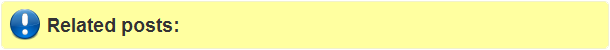## Friday, May 21, 2010

### Parallel geoprocessing in ArcGIS Desktop.

You will need to edit the Python script supplied to support concurrent geoprocessing of multiple processes. To make these edits you will need an understanding of how to script geoprocessing using Python.

Be aware this is just an example of an approach to parallelization of geoprocessing, there may be no benefit in the approach in your particular environment or task.

# Author: ESRI
# Date: August 2009
# Purpose: This script demonstrates a methodology for parallel geoprocessing in ArcGIS Desktop.
# The input is split into parts which are processed by separate subprocesses,
# their outputs are appended into the final result. In this specific case, the
# geoprocessing undertaken is address geocoding, but any feasible geoprocessing
# can be done by simple modification of this script.
# Look for the comment line "Begin code block for GP process of interest"

try:
import arcgisscripting, os, subprocess, sys, time, traceback
gp = arcgisscripting.create(9.3)
gp.overwriteoutput = True
startTime = time.time()
#Get the number of concurrent GP processes desired.
#It is recommended this agree with the number of your available cores.
pCount = gp.getparameter(0)

inObject = gp.getparameterastext(1)
result = gp.getcount_management(inObject)
count = int(result.getoutput(0))
gp.addmessage("Processing " + inObject + " in " + str(pCount) + " concurrent processes.")

#We will use a generic approach to splitting the input into parts, namely slicing it
#with modulo arithmetic. For example, if we want to run 4 concurrent processes
#we create 4 parts from the input using "ObjectID modulo 4 = 0 (then 1,2,3)"
#Build the splitting expressions...(Note you will need to edit this code block if using input from SDE on Oracle)
inDesc = gp.describe(inObject)
wsType = str(gp.describe(str(inDesc.path)).workspacetype)
queryList = []
for q in range(pCount):
if wsType == "RemoteDatabase": #SDE
#Non-Oracle
queryList.append(delimitedOIDName + " % " + str(pCount) + " = " + str(q))
#Oracle - comment out the above line and uncomment the below line when using Oracle inputs
#queryList.append("mod(\"" + delimitedOIDName + "\"," + str(pCount) + ") = " + str(q))
elif wsType == "FileSystem": #DBase
queryList.append("mod(\"" + delimitedOIDName + "\"," + str(pCount) + ") = " + str(q))
elif wsType == "LocalDatabase" and inDesc.path.endswith(".mdb"): #Personal GDB
queryList.append(delimitedOIDName + " mod " + str(pCount) + " = " + str(q))
elif wsType == "LocalDatabase" and inDesc.path.endswith(".gdb"): #File GDB
queryList.append("mod(\"" + delimitedOIDName + "\"," + str(pCount) + ") = " + str(q))

#Create a seed name for the parts
if inDesc.name.endswith((".dbf",".shp")):
seedName = str(inDesc.name).split(".")
elif inDesc.name.count(".") > 1: #Fully qualified DBMS object name
seedName = str(inDesc.name).split(".")[-1]
else:
seedName = str(inDesc.name)
#Make file GDBs at a well known path (to the system); edit this to use fast disk if you have it.
#The child scripts will write into these separate file GDBs so as to avoid lock conflicts.
#import tempfile
#wkPath = tempfile.gettempdir()
wkPath = r"C:\Temp"
gdbNameList = []
j = 0
for i in range(pCount):
gdbName = wkPath+"\parallel"+str(i)+".gdb"
while gp.exists(gdbName): #Do not clobber other parallel processes
j+=1
gdbName = wkPath+"\parallel"+str(j)+".gdb"
gdbNameList.append(gdbName)
result = gp.createfilegdb_management(wkPath,os.path.basename(gdbName))
gp.workspace = "in_memory"
#Create scripts that geoprocess the parts, write these into the file GDB directories created above
scriptPathList = []
for r in range(pCount):
gdbName = gdbNameList[r]
sName = gdbName+"/parallel.py"
scriptPathList.append(sName)
scriptLines = []
scriptLines.append("import arcgisscripting\n")
scriptLines.append("gp = arcgisscripting.create(9.3)\n")
scriptLines.append("gp.overwriteoutput = True\n")
if str(inDesc.datatype) == "Table" or str(inDesc.datatype) == "DbaseTable":
scriptLines.append("result = gp.tabletotable_conversion("+\
"\""+inObject+"\","+\
"\""+gdbName+"\","+\
"\""+seedName+str(r)+"\","+\
"\""+queryList[r]+"\")\n")
else:
scriptlines.append("result = gp.featureclasstofeatureclass_conversion("+\
"\""+inObject+"\","+\
"\""+gdbName+"\","+\
"\""+seedName+str(r)+"\","+\
"\""+queryList[r]+"\")\n")
scriptLines.append("part = result.getoutput(0)\n")
#
#Begin code block for GP process of interest:
scriptLines.append("aLocator = r\"C:\Data\Work\Product Management\Geoprocessing\TestData.gdb\NZ Locator\""+"\n")
#We could use a service. Note the \\a in \\arcgis below would be a BELL literal if not escaped as in \a (rcgis)
#scriptLines.append("aLocator = r\"GIS Servers\\arcgis on bruceh\CA_Locator.GeocodeServer\""+"\n")
scriptLines.append("outFC = "+"\""+gdbName+"\\"+"Result"+str(r)+"\"\n")
scriptLines.append("result = result.getoutput(0)\n")
#End code block for GP process of interest:
#
#Make stdio aware of completion - this message will be returned to the GP stdio message pipe
scriptLines.append("print "+ "\"Finished Part" + str(r) + "\"\n")
scriptLines.append("del gp\n")
#Write script
f = open(sName,'w')
f.writelines(scriptLines)
f.flush
f.close()

#Launch the parallel processes
processList = []
for r in range(pCount):
processList.append(subprocess.Popen([r"C:\Python25\python.exe",scriptPathList[r]],\
shell=True,\
stdout=subprocess.PIPE,\
stderr=subprocess.PIPE))
gp.addmessage("Launched process " + str(r)+ "\n")
time.sleep(2)
#
#Wait for completion
messageList = []
Done = False
while not Done:
Done = True
for p in processList:
if p.poll() is None:
Done = False
else:
messageList.append("Process " + str(processList.index(p)) + " complete at " + str(time.ctime()) + "\n")
for s in stdOutLines:
messageList.append(s)
for e in stdErrorLines:
messageList.append(e)
if not Done: #Save 2 seconds if complete...
time.sleep(2)
for m in messageList:

#Merge the results
outFC = gp.getparameterastext(2)
fm = gp.createobject("fieldmappings")
vt = gp.createobject("valuetable")
for g in gdbNameList:
gp.workspace = g
if len(gp.listfeatureclasses("Result*")) > 0:
fcDesc = gp.describe(gp.listfeatureclasses("Result*"))
gp.workspace = "in_memory"
result = gp.merge_management(vt,outFC,fm)
#Clean up
for g in gdbNameList:
result = gp.delete_management(g)
#Done!
endTime = time.time()
gp.addmessage("Geoprocessing duration was " + str(int(endTime - startTime)) + " seconds.")
gp.addmessage("Net performance was " + \
str(int(1.0 * count / (endTime - startTime) * 3600.0)) + " records per hour.")
del gp
except:
tb = sys.exc_info()
tbinfo = traceback.format_tb(tb)
pymsg = "PYTHON ERRORS:\nTraceback Info:\n" + tbinfo + "\nError Info:\n " + \
str(sys.exc_type)+ ": " + str(sys.exc_value) + "\n"